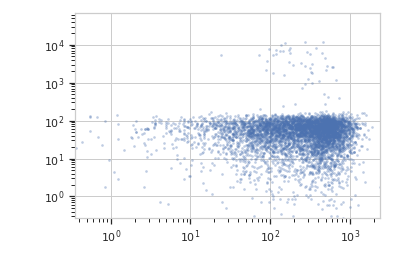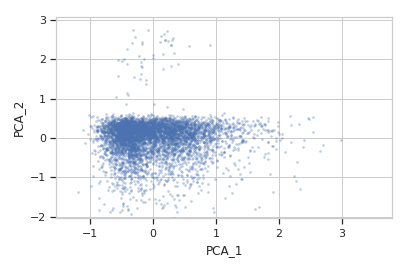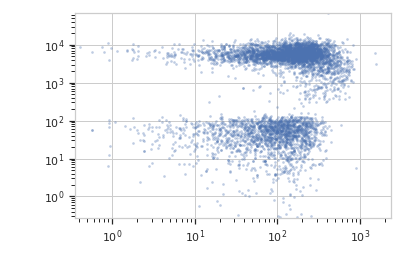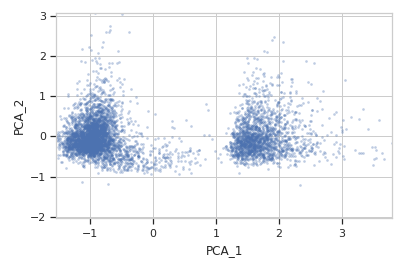# cytoflow.operations.pca¶

class cytoflow.operations.pca.PCAOp[source]

Use principal components analysis (PCA) to decompose a multivariate data set into orthogonal components that explain a maximum amount of variance.

Call estimate() to compute the optimal decomposition.

Calling apply() creates new “channels” named {name}_1 ... {name}_n, where name is the name attribute and n is num_components.

The same decomposition may not be appropriate for different subsets of the data set. If this is the case, you can use the by attribute to specify metadata by which to aggregate the data before estimating (and applying) a model. The PCA parameters such as the number of components and the kernel are the same across each subset, though.

name

The operation name; determines the name of the new columns.

Type: Str
channels

The channels to apply the decomposition to.

Type: List(Str)
scale

Re-scale the data in the specified channels before fitting. If a channel is in channels but not in scale, the current package-wide default (set with set_default_scale()) is used.

Type: Dict(Str : {“linear”, “logicle”, “log”})
num_components

How many components to fit to the data? Must be a positive integer.

Type: Int (default = 2)
by

A list of metadata attributes to aggregate the data before estimating the model. For example, if the experiment has two pieces of metadata, Time and Dox, setting by to ["Time", "Dox"] will fit the model separately to each subset of the data with a unique combination of Time and Dox.

Type: List(Str)
whiten

Scale each component to unit variance? May be useful if you will be using unsupervized clustering (such as K-means).

Type: Bool (default = False)

Examples

Make a little data set.

>>> import cytoflow as flow
>>> import_op = flow.ImportOp()
>>> import_op.tubes = [flow.Tube(file = "Plate01/RFP_Well_A3.fcs",
...                              conditions = {'Dox' : 10.0}),
...                    flow.Tube(file = "Plate01/CFP_Well_A4.fcs",
...                              conditions = {'Dox' : 1.0})]
>>> import_op.conditions = {'Dox' : 'float'}
>>> ex = import_op.apply()


Create and parameterize the operation.

>>> pca = flow.PCAOp(name = 'PCA',
...                  channels = ['V2-A', 'V2-H', 'Y2-A', 'Y2-H'],
...                  scale = {'V2-A' : 'log',
...                           'V2-H' : 'log',
...                           'Y2-A' : 'log',
...                           'Y2-H' : 'log'},
...                  num_components = 2,
...                  by = ["Dox"])


Estimate the decomposition

>>> pca.estimate(ex)


Apply the operation

>>> ex2 = pca.apply(ex)


Plot a scatterplot of the PCA. Compare to a scatterplot of the underlying channels.

>>> flow.ScatterplotView(xchannel = "V2-A",
...                      xscale = "log",
...                      ychannel = "Y2-A",
...                      yscale = "log",
...                      subset = "Dox == 1.0").plot(ex2)

>>> flow.ScatterplotView(xchannel = "PCA_1",
...                      ychannel = "PCA_2",
...                      subset = "Dox == 1.0").plot(ex2)>>> flow.ScatterplotView(xchannel = "V2-A",
...                      xscale = "log",
...                      ychannel = "Y2-A",
...                      yscale = "log",
...                      subset = "Dox == 10.0").plot(ex2)

>>> flow.ScatterplotView(xchannel = "PCA_1",
...                      ychannel = "PCA_2",
...                      subset = "Dox == 10.0").plot(ex2)estimate(experiment, subset=None)[source]

Estimate the decomposition

Parameters: experiment (Experiment) – The Experiment to use to estimate the k-means clusters subset (str (default = None)) – A Python expression that specifies a subset of the data in experiment to use to parameterize the operation.
apply(experiment)[source]

Apply the PCA decomposition to the data.

Returns: a new Experiment with additional channels named name_1 ... name_n Experiment